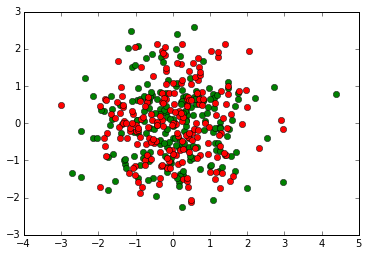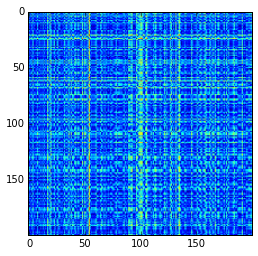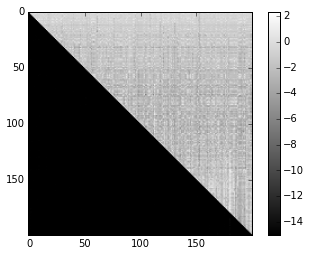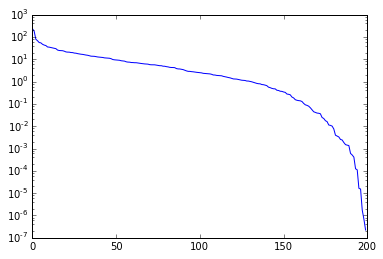# Rank of a Potential Evaluation Matrix¶

In :
import numpy as np
import matplotlib.pyplot as pt


Let's make two particle collections: sources and targets

In :
sources = np.random.randn(2, 200)
targets = np.random.randn(2, 200)

pt.plot(sources, sources, "go")
pt.plot(targets, targets, "ro")

Out:
[<matplotlib.lines.Line2D at 0x7f7914197e10>]Now let's assume each of these points has a charge, and evaluate the potential at each of the other points.

In :
all_distvecs = sources.reshape(2, 1, -1) - targets.reshape(2, -1, 1)
dists = np.sqrt(np.sum(all_distvecs**2, axis=0))
interaction_mat = 1/dists

pt.imshow(dists)

Out:
<matplotlib.image.AxesImage at 0x7f790fe6d198>## Finding the Rank: Attempt 1¶

How do we find the rank? Get the matrix to echelon form, look for zero rows.

Bonus Q: Is this the same as LU?

In :
from m_echelon import m_echelon
M, U = m_echelon(interaction_mat)
pt.imshow(np.log10(1e-15+np.abs(U)), cmap="gray")
pt.colorbar()

Out:
<matplotlib.colorbar.Colorbar at 0x7f790fd858d0>## Finding the Rank: Attempt 2¶

In :
U, sigma, V = np.linalg.svd(interaction_mat)

pt.semilogy(sigma)

Out:
[<matplotlib.lines.Line2D at 0x7f790ffdf908>]In :
k = 60
Uk = U[:, :k]
Vk = V.T[:, :k].T

Ak = (Uk * sigma[:k]).dot(Vk)

np.linalg.norm(interaction_mat - Ak, 2)

Out:
7.0952237471847974
In :